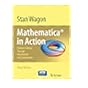Normal view

# Mathematica in Action [electronic resource] : Problem Solving Through Visualization and Computation / edited by Stan Wagon.

Material type:TextPublisher: New York, NY : Springer New York : Imprint: Springer, 2010Edition: 3Description: XI, 580 p. online resourceContent type: text Media type: computer Carrier type: online resourceISBN: 9780387754772Additional physical formats: Printed edition:: No titleDDC classification: 518 LOC classification: QA71-90Online resources: Click here to access online
Contents:
A Brief Introduction -- Plotting -- Prime Numbers -- Rolling Circles -- Three-Dimensional Graphs -- Dynamic Manipulations -- The Cantor Set, Real and Complex -- The Quadratic Map -- The Recursive Turtle -- Parametric Plotting of Surfaces -- Penrose Tiles -- Complex Dynamics:Julia Sets and the Mandelbrot Set -- Solving Equations -- Optimization -- Differential Equations -- Computational Geometry -- Check Digits and the Pentagon -- Coloring Planar Maps -- New Directions For ? -- The Banach–Tarski Paradox -- The Riemann Zeta Function -- Miscellany.
Summary: In this third edition of Mathematica® in Action, award-winning author Stan Wagon guides beginner and veteran users alike through Mathematica's powerful tools for mathematical exploration. The transition to Mathematica 7 is made smooth with plenty of examples and case studies that utilize Mathematica's newest tools, such as dynamic manipulations and adaptive three-dimensional plotting. Mathematica in Action also emphasizes the breadth of Mathematica and the impressive results of combining techniques from different areas. This material enables the reader to use Mathematica to solve a variety of complex problems. Case studies ranging from elementary to sophisticated are provided throughout. Whenever possible, the book shows how Mathematica can be used to discover new things. Striking examples include the design of a road on which a square wheel bike can ride, the design of a drill that can drill square holes, an illustration of the Banach—Tarski Paradox via hyperbolic geometry, new and surprising formulas for p, the discovery of shadow orbits for chaotic systems, and the use of powerful new capabilities for three-dimensional graphics. Visualization is emphasized throughout, with finely crafted graphics in each chapter. Wagon is the author of eleven books on mathematics, including A Course in Computational Number Theory, named one of the ten best math books of 2000 by the American Library Association. He has written extensively on the educational applications of Mathematica, including the books VisualDSolve: Visualizing Differential Equations with Mathematica, and Animating Calculus: Mathematica Notebooks for the Laboratory. From reviews of the second edition: "In a dazzling range of examples Stan Wagon shows how such features as animation, 3-dimensional graphics and high-precision integer arithmetic can contribute to our understanding and enjoyment of mathematics." —Richard Walker, The Mathematical Gazette "The bottom line is that Mathematica in Action is an outstanding book containing many examples of real uses of Mathematica for the novice, intermediate, and expert user." —Mark McClure, Mathematica in Education and Research.
Tags from this library: No tags from this library for this title.Average rating: 0.0 (0 votes)
Item type Current location Collection Call number Status Date due Barcode Item holdseBook e-Library

Electronic Book@IST

EBook Available
Total holds: 0

A Brief Introduction -- Plotting -- Prime Numbers -- Rolling Circles -- Three-Dimensional Graphs -- Dynamic Manipulations -- The Cantor Set, Real and Complex -- The Quadratic Map -- The Recursive Turtle -- Parametric Plotting of Surfaces -- Penrose Tiles -- Complex Dynamics:Julia Sets and the Mandelbrot Set -- Solving Equations -- Optimization -- Differential Equations -- Computational Geometry -- Check Digits and the Pentagon -- Coloring Planar Maps -- New Directions For ? -- The Banach–Tarski Paradox -- The Riemann Zeta Function -- Miscellany.

In this third edition of Mathematica® in Action, award-winning author Stan Wagon guides beginner and veteran users alike through Mathematica's powerful tools for mathematical exploration. The transition to Mathematica 7 is made smooth with plenty of examples and case studies that utilize Mathematica's newest tools, such as dynamic manipulations and adaptive three-dimensional plotting. Mathematica in Action also emphasizes the breadth of Mathematica and the impressive results of combining techniques from different areas. This material enables the reader to use Mathematica to solve a variety of complex problems. Case studies ranging from elementary to sophisticated are provided throughout. Whenever possible, the book shows how Mathematica can be used to discover new things. Striking examples include the design of a road on which a square wheel bike can ride, the design of a drill that can drill square holes, an illustration of the Banach—Tarski Paradox via hyperbolic geometry, new and surprising formulas for p, the discovery of shadow orbits for chaotic systems, and the use of powerful new capabilities for three-dimensional graphics. Visualization is emphasized throughout, with finely crafted graphics in each chapter. Wagon is the author of eleven books on mathematics, including A Course in Computational Number Theory, named one of the ten best math books of 2000 by the American Library Association. He has written extensively on the educational applications of Mathematica, including the books VisualDSolve: Visualizing Differential Equations with Mathematica, and Animating Calculus: Mathematica Notebooks for the Laboratory. From reviews of the second edition: "In a dazzling range of examples Stan Wagon shows how such features as animation, 3-dimensional graphics and high-precision integer arithmetic can contribute to our understanding and enjoyment of mathematics." —Richard Walker, The Mathematical Gazette "The bottom line is that Mathematica in Action is an outstanding book containing many examples of real uses of Mathematica for the novice, intermediate, and expert user." —Mark McClure, Mathematica in Education and Research.

There are no comments for this item.Mathematica® in Action: Problem Solving Through Visualization and Computation. by Wagon, Stan. ©2010